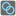• # Help

## Question related to missionBreak Rings

Hi guys I stuck here

```from collections import Counter
r=list(rings)
count=0

while r:
d=Counter(x for sublist in r for x in sublist)
a=d.most_common()
d=a
for i in r[:]:
if d in i:
r.remove(i)
count+=1
return count
```

can't pass extra test 8 Any help???

I also try another solution but same thing

```    from collections import Counter
r = list (({3,4},{5,6},{2,7},{1,5},{2,6},{8,4},{1,7},{4,5},{9,5},{2,3},{8,2},{2,4},{9,6},{5,7},{3,6},{1,3},))
count=0
count2=0
c=list(r)
while r:
d=Counter(x for sublist in r for x in sublist)
a=d.most_common()
d=a
for i in r[:]:
if d in i:
r.remove(i)
count+=1
while c:
d=Counter(x for sublist in c for x in sublist)
a=d.most_common()
d=a
if a ==a :
d=a
for i in c[:]:
if d in i:
c.remove(i)
count2+=1

if count > count2:
print(count)
else:
print(count2)
```11